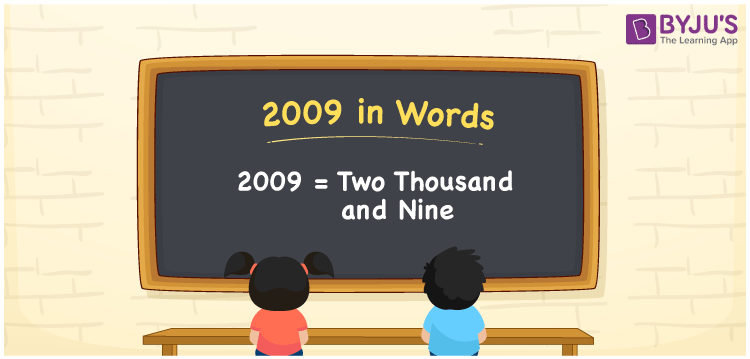# 2009 in Words

2009 in words is “Two Thousand and Nine”. In mathematics, the number 2009 is a cardinal number, which helps to express something. For example, Meera was born in 2009. To convert 2009 into words, we will use the place value system. This article will guide you on the procedure of writing 2009 in words with a complete explanation.

 2009 in Words: Two Thousand and Nine. Two Thousand and Nine in Numerical Form: 2009.

## 2009 in English Words## How to Write 2009 in Words?

We will use the place value system for writing the number 2009 in words. Go through the below table and learn the place values of 2009.

 Thousands Hundreds Tens Ones 2 0 0 9

The expanded form of 2009 is as follows:

= 2 × Thousand + 0 × Hundred + 0 × Ten + 9 × One

= 2 × 1000 + 0 × 100 + 0 × 10 + 9 × 1

= 2000 + 9

= 2009

= two thousand and nine

Hence, 2009 in words is Two thousand and nine.

2009 in words – Two thousand and nine

Is 2009 an odd number? – Yes

Is 2009 an even number? – No

Is 2009 a perfect square number? – No

Is 2009 a perfect cube number? – No

Is 2009 a prime number? – No

Is 2009 a composite number? – Yes

## Frequently Asked Questions on 2009 in Words

Q1

### How to write 2009 in words?

2009 in words is two thousand and nine.

Q2

### Simplify 2000 + 9, and express it in words.

Simplifying 2000 + 9, we get 2009. Hence, 2009 in words is two thousand and nine.

Q3

### Is 2009 an odd number?

Yes, 2009 is an odd number.